# hw5S17 .pdf

### File information

Original filename: hw5S17.pdf

This PDF 1.5 document has been generated by LaTeX with hyperref package / pdfTeX-1.40.16, and has been sent on pdf-archive.com on 03/03/2017 at 20:04, from IP address 137.99.x.x. The current document download page has been viewed 392 times.
File size: 158 KB (5 pages).
Privacy: public file

hw5S17.pdf (PDF, 158 KB)

### Document preview

CSE4102: Programming Languages

Spring 2017

Homework 5: Lambda Calculus Interpreter
Out Date:
Due Date:

03/01/2017
03/09/2017

Objectives
The purpose of this assignment is to produce a complete lambda calculus interpreter in ML. To make your
task easier, we provide three SML source files on the moodle site. Submit a zip file with your answers. Do
respect the name of the functions to ease grading. The problem statements are pretty short and the answers
are even shorter. You start with a set implementation as well as a handful of convenience functions to
work with. In particular, we provide, in church.sml, a structure with the implementation of two functions
to convert natural numbers into Church numerals. Namely, given the number 3, the function int2Church
will produce λf.λs.f (f (f s)). Similarly, the function church2Int will turn λf.λs.f (f (f s)) (or any alphaequivalent expression) back into the natural number 3. Similarly, we provide a set abstract data type
implementation in the set.sml file. The ADT is pretty straightforward and uses a list to represent a set.
The API is simply
signature S e t = s i g
type ’ ’ a S e t
val s i n g l e : ’ ’ a −&gt; ’ ’ a S e t
val empty : u n i t −&gt; ’ ’ a S e t
val i n s e r t : ’ ’ a −&gt; ’ ’ a S e t −&gt; ’ ’ a S e t
val union : ’ ’ a S e t −&gt; ’ ’ a S e t −&gt; ’ ’ a S e t
val i n t e r
: ’ ’ a S e t −&gt; ’ ’ a S e t −&gt; ’ ’ a S e t
val member : ’ ’ a −&gt; ’ ’ a S e t −&gt; b o o l
val remove : ’ ’ a −&gt; ’ ’ a S e t −&gt; ’ ’ a S e t
val show
: ’ ’ a S e t −&gt; ( ’ ’ a −&gt; s t r i n g ) −&gt; s t r i n g
end ;
Namely, you can:
single x create a singleton with a value x of type ”a
empty create an empty set
insert a b add a value a of type ”a into a set b. Return the new set.
union a b produce a new set equal to the union of the two input sets, i.e., return a ∪ b.
inter a b produce a new set equal to the intersection of the two input sets, i.e., return a ∩ b.
member a b returns true if an element a (of type ”a) belongs to set b, i.e., return a ∈ b.
remove a b returns a new set identical to the input set b except that the provided element a no longer
appears in the set, i.e., return b \ {a}.
show b f given a set b and an element printing function f , produce a string that corresponds to the content
of the set.
The assignment is staggered and parts should be solved in order.

1

Question 0
For this question, load the file lambda.sml. A lambda expression is defined as a value that belongs to the
datatype expr defined in lambda.sml. For instance, the function λx.x that denotes the identity function is
encoded with the SML value
val i d f = Lambda . abs ( ”x” , Lambda . var ( ”x” ) )
Clearly, Lambda.var refers to the occurrence of a variable within the body of an expression and Lambda.abs(v,b)
is an SML constructor that defines an abstraction (function) over variable v and with a body b. A function
call such as (λx.x)y would be:
val l c = Lambda . apply ( Lambda . abs ( ”x” , Lambda . var ( ”x” ) ) , var ( ”y” ) )
in which Lambda.apply(a,b) is the SML constructor for a lambda calculus function call where a is the
function and b the argument.
Within the Lambda structure, write a function freeV which, given a lambda calculus expression e returns
the set of free variables in e. Namely, it should produce a set of strings where each string is the identifier
of a non-bound variable in e. The definition should, of course, be inductive based on the structure of e. On
the example
(λx.x)y
encoded as
val l c = Lambda . apply ( Lambda . abs ( ”x” , Lambda . var ( ”x” ) ) , var ( ”y” ) )
A call (freeV lc) should return the set
{”y”}

Question 1
In the same lambda.sml and the same Lambda structure, write an SML function newName which, given
a set of strings S, produces a new unique string that does not belong to S. For instance from the set
S = {00 x00 ,00 y 00 ,00 z 00 ,00 x000 ,00 x100 }, newName could produce “x2” or “y1” or even “w” as none of these strings
appear in S.

2

Question 2
Consider the following signature defining Lambda expressions:
signature LExpr = s i g
exception Bad of s t r i n g
datatype expr = var
of s t r i n g
| apply of expr
∗ expr
| abs
of s t r i n g ∗ expr
type ’ a S e t
val t o S t r i n g : expr −&gt; s t r i n g
val f r e e V
: expr −&gt; s t r i n g S e t
val newName : s t r i n g S e t −&gt; s t r i n g
val
val
val
val
val

subst
alpha
normal
beta
simp

:
:
:
:
:

s t r i n g −&gt; expr −&gt; expr −&gt; expr
expr −&gt; s t r i n g S e t −&gt; expr
expr −&gt; b o o l
expr −&gt; expr
expr −&gt; expr

end
You already implemented freeV and newName in the previous question. It is now time to implement the
five remaining functions that produce a complete lambda-calculus interpreter. To refresh your memory,
the functions are described below. Naturally, feel free to refer to the slides for more details. Note how all
functions adopt the curry style (and you are expected to conform to this requirement).
subst This simple function takes three arguments x,a,b and implement a substitution [a/x]b as described in
the notes. Namely, it replaces every occurrence of x appearing within b by expression a. The function
is not concerned by accidental bindings and blindly does the replacement.
alpha The alpha function takes a lambda expression e and a set P of prohibited identifiers and its role is to
rename the binders in expression e to avoid accidental bindings that would occur as a result of doing
a beta reduction with arguments containing free variables whose names appear in P .
beta The beta function is responsible for a one step reduction through a beta reduction. Namely, it takes
as input an expression e which is not in normal form and finds, within e, a site of the form e =
α((λx.body)arg)γ, namely, it finds an application where the left expression is a function. In that case,
this can be reduced as
α ((λx.body)arg) γ →β α ([arg/x]body) γ
provided that x does not occur freely in arg (if it does, one should first α-reduce).
normal The normal function takes as input a lambda expression e and determines whether that expression
is irreducible or not. If the function contains a site with a reducible beta, then it should return false.
Otherwise it should returns true. You
simp This final function takes as input a lambda expression e and is responsible for repeatedly rewriting e
though a beta reduction until the expression is in normal form. Clearly, this is your top-level interpreter.

Question 3
Once you have these five functions, you are ready to test your interpreter. To do so, you must write several
lambda programs of increasing complexity, all the way to a recursive implementation of the classic fibonacci

3

function. To get you started, we provide two lambda structures. One is a ’demo’ of how to
test addition (plus lambda expression) the other is the skeleton for building your solution to
the extra-credit Question 4. All this code is in expr.sml For instance, to define a piece of code that
defines lambda expressions and strings them together into a program, one could write for the expressions:
Y
T
F

= λf.(λx.f (xx))(λx.f (xx))
= λx.λy.x
= λx.λy.y

The SML code shown below
structure Main = struct
structure L : LExpr = Lambda ;
structure C : ChurchSig = Church ;
fun f i b v a l u e =
l e t open L
in l e t val t = abs ( ”x” , apply ( var ” f ” , apply ( var ”x” , var ”x” ) ) )
val Y = abs ( ” f ” , apply ( t , t ) )
val tlam = abs ( ”x” , abs ( ”y” , var ”x” ) )
val flam = abs ( ”x” , abs ( ”y” , var ”y” ) )
...
in . . .
end
end
which would have to be extended to include all the necessary fragments. For this question, The lambda
expression you are expected to write and test include
succ λn.λs.λz.s(n s z) which computes the successor of n given as a church numeral.
isZero λn.n(λx.λa.λb.b)(λa.λb.a) which, given a number n outputs true is n = 0 and false otherwise.
plus λn.λm.n succ m which computes the sum of two non-negative numbers (given as church numerals)
mult λn.λm.λf.n(mf ) which computes the product of two church numerals n and m.
pair λa.λb.λz.zab which creates from two lambda expressions a and b, a lambda expression for the pair
(a, b)
first λp.p(λa.λb.a) which, given a pair p returns the first element of the pair.
second λp.p(λa.λb.b) which, given a pair p returns the second element of the pair.
pred A lambda expression (check the notes) which given a church numeral n computes the predecessor of
n, i.e., n − 1.

4

For Each lambda expression above, create a test that feeds a value and produces the output. For instance:
structure Main = struct
structure L : LExpr = Lambda ;
structure C : ChurchSig = Church ;
fun t e s t 3 ( ) = (∗ This i s t h e t e s t f u n c t i o n f o r t h e 3 rd e x p r . −− p l u s −− ∗)
l e t open L in
l e t val t = abs ( ”x” , apply ( var ” f ” , apply ( var ”x” , var ”x” ) ) )
val Y = abs ( ” f ” , apply ( t , t ) )
val s u c c = . . .
val i s Z e r o = . . .
val p l u s = . . .
val mult = . . .
val p a i r = . . .
val f i r s t = . . .
val s e c o n d = . . .
val pred = . . .
val t o T e s t = apply ( apply ( p l u s , ( C . int2Church 2 ) ) , (C . int2Church 3 ) )
in p r i n t ( t o S t r i n g ( simp t o T e s t ) ˆ ” \n” )
end
end
end
should evaluate to the church numeral for 5 (up to alpha-renaming) and print it out. You are supposed
to write one test for each lambda expression (8 in total as listed above). Note that the sample code
provides the test for the plus expression. Thus, the bulk of the work is to write the lambda expression
and test them.

Question 4: Extra Credit 100%
(Yes, you read this correctly: if you did great so far, you can double the value of this homework. Note that
there is no point attempting the extra credit if you do not have a functioning interpreter from question 3.)
Finally, consider the classic recursive Fibonacci function whose specification is given as
fun f i b 0 = 0
| fib 1 = 1
| f i b n = f i b ( n−1) + f i b ( n−2)
Implement it as a lambda expression and use the Y combinator. Test it on a value (e.g., 7) as follows
structure L : LExpr = Lambda ;
fun l f i b n = l e t open L in
l e t val expr = apply ( apply (Y, f i b E x p r ) , Church . int2Church n )
in p r i n t ( t o S t r i n g ( simp expr ) ˆ ” \n” )
end
end
lfib 7
Note how this code creates a big lambda expression and relies on the simp routine of the Lambda structure
to evaluate the program.

Have fun!
5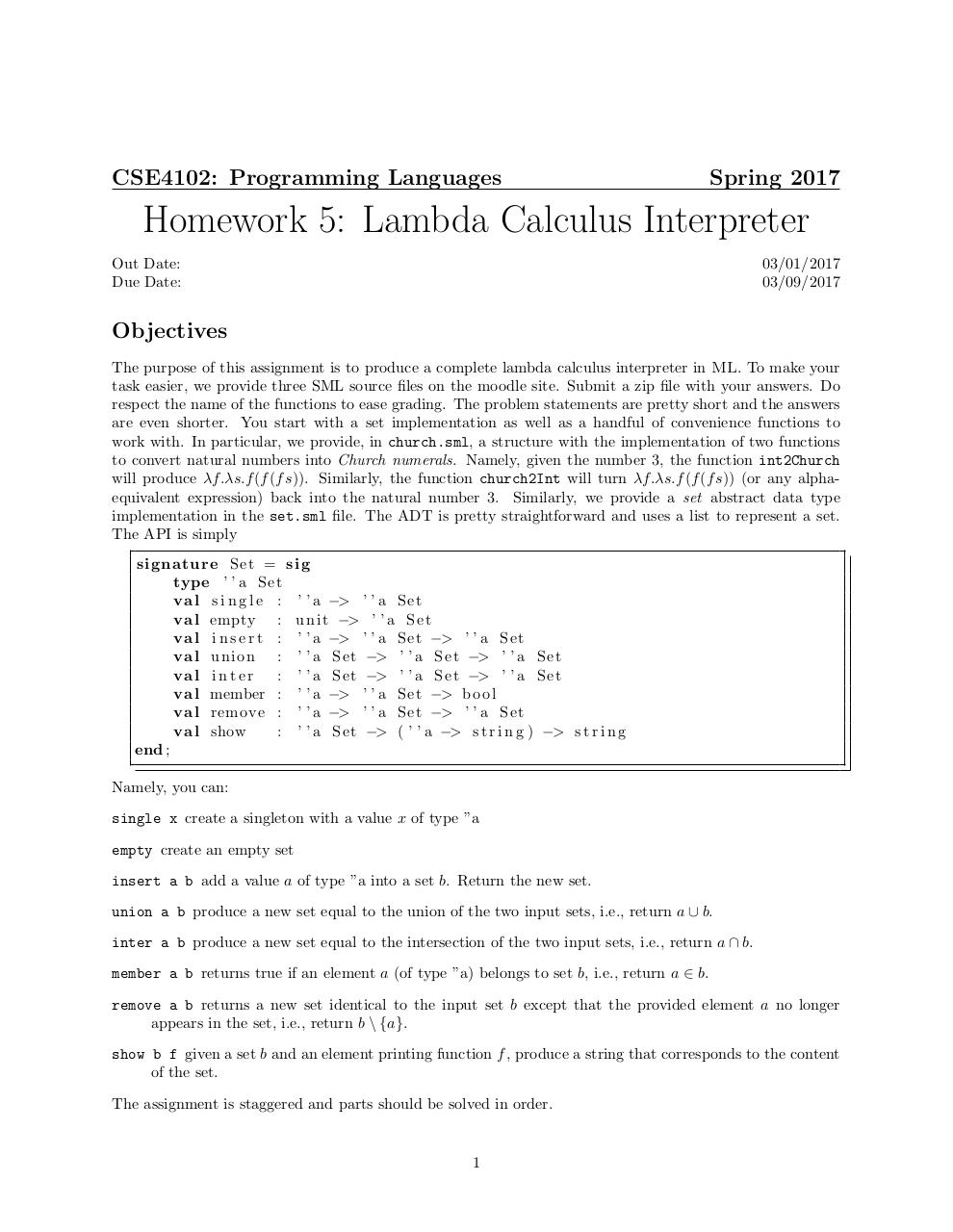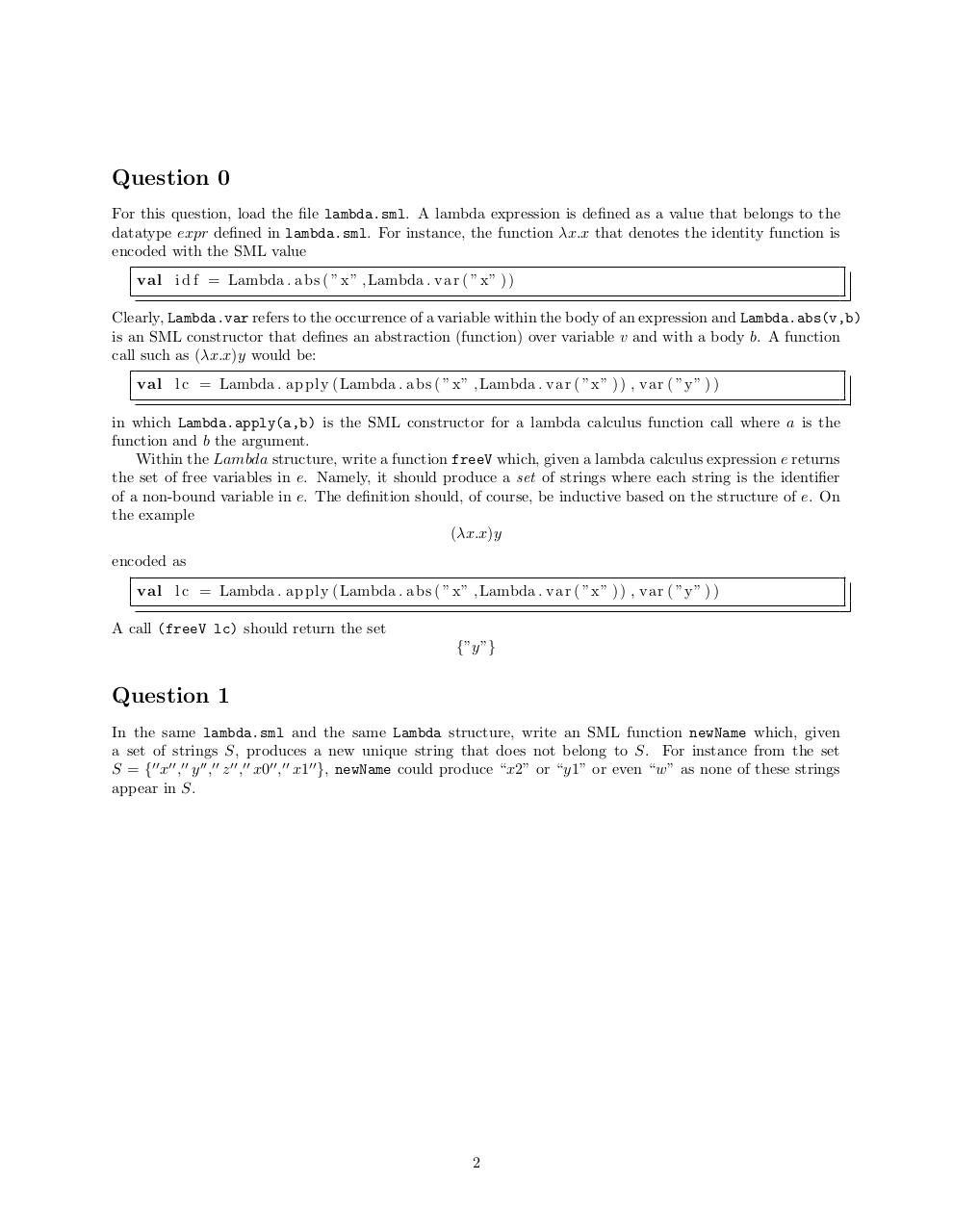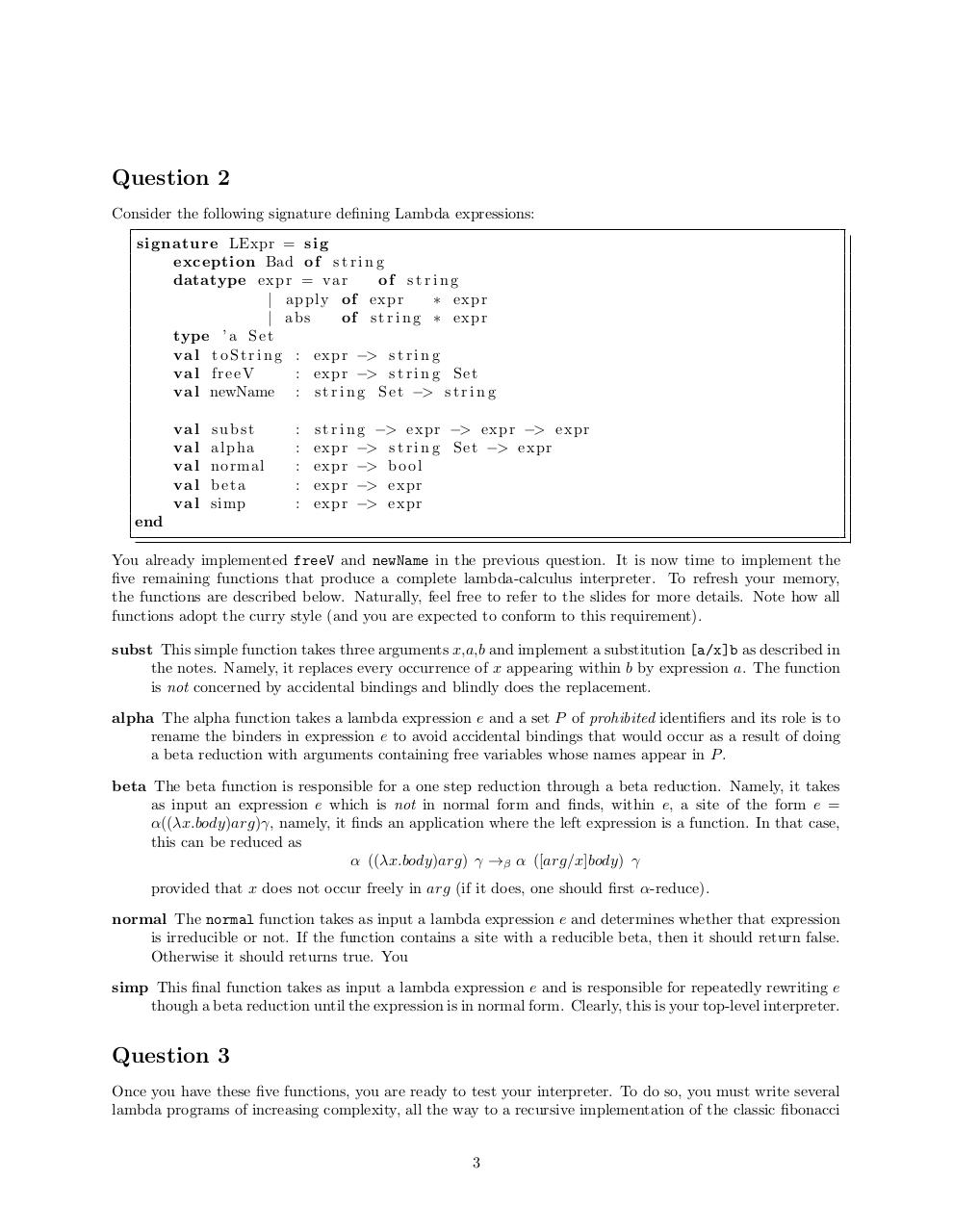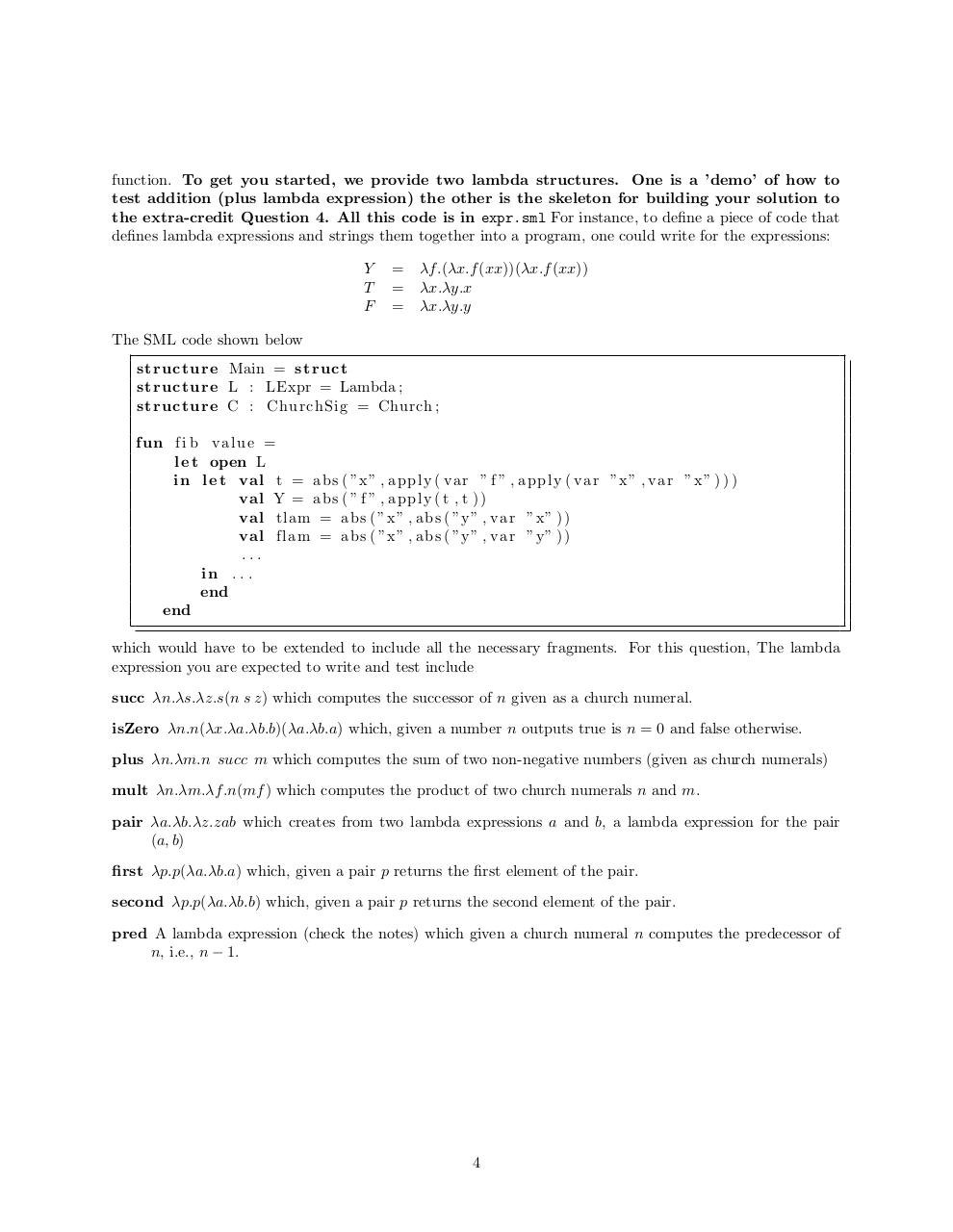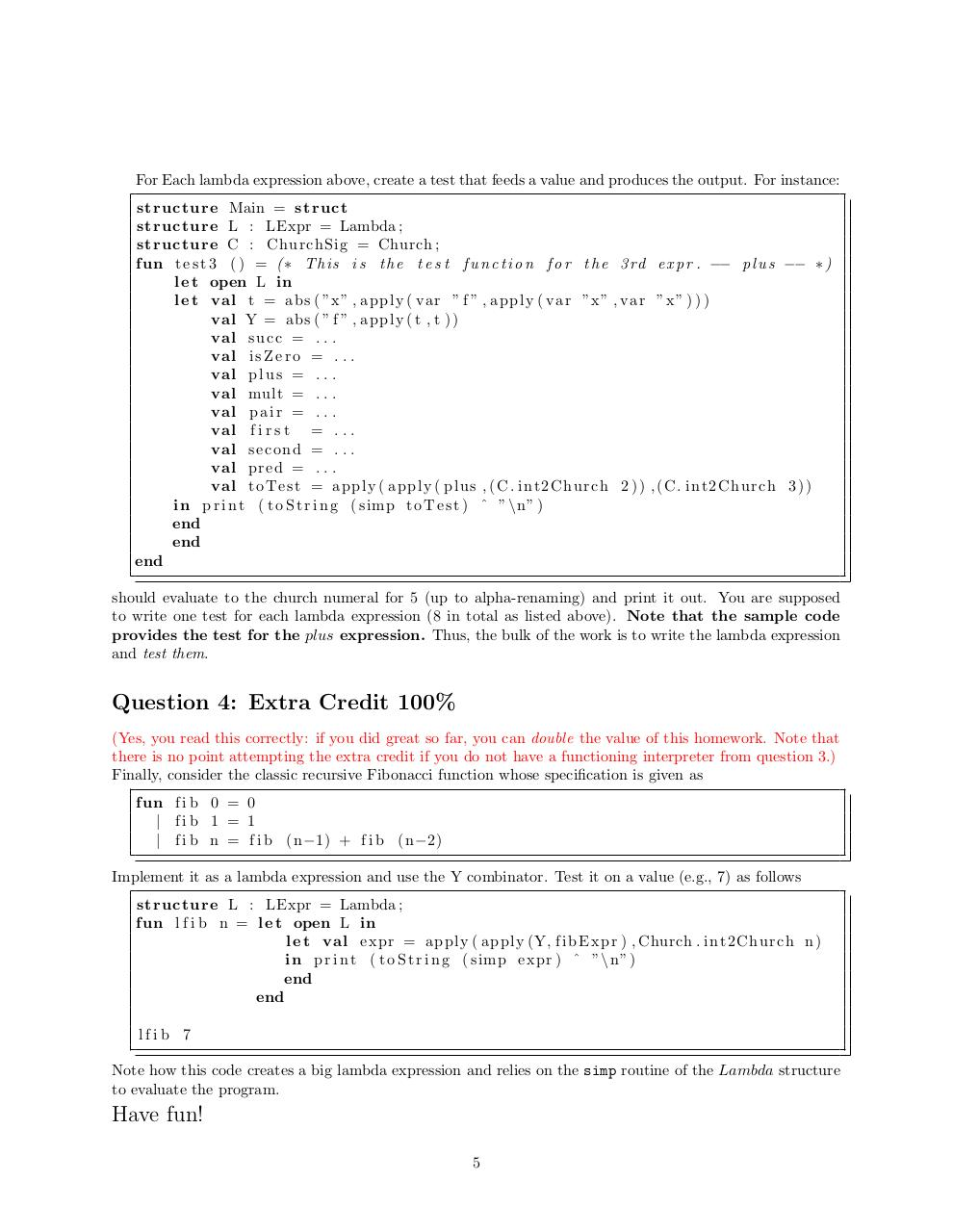#### HTML Code

Copy the following HTML code to share your document on a Website or Blog

#### QR Code### Related keywords